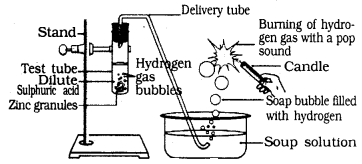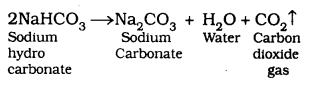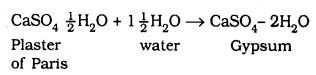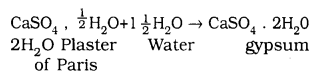# KSEEB SSLC Class 10 Science Solutions Chapter 2 Acids, Bases and Salts

KSEEB SSLC Class 10 Science Solutions Chapter 2 Acids, Bases and Salts are part of KSEEB SSLC Class 10 Science Solutions. Here we have given Karnataka SSLC Class 10 Science Solutions Chapter 2 Acids, Bases and Salts.

## Karnataka SSLC Class 10 Science Solutions Chapter 2 Acids, Bases and Salts

### KSEEB SSLC Class 10 Science Chapter 1 Intext Questions

Text Book Part I Page No. 18

KSEEB Solutions For Class 10 Science Acid Base And Salt Question 1.
You have been provided with three test tubes. One of them contains distilled water and the other two contain an acidic solution and a basic solution, respectively. If you are given only red litmus paper, how will you identify the contents of each test tube?
Answer:

We can identify the contents of each test tube, with the help of red litmus paper. Consider the following observations:

1. If there is little change in the colour of red litmus (such as purple), then it is distilled water.
2. If the colour of red litmus remains the same, then it is acid.
3. If the colour of red litmus becomes blue, then it is base.

Text Book Part I Page No. 22

KSEEB Solutions For Class 10 Science Chapter 2 Question 1.
Why should curd and sour substances not be kept in brass and copper vessels?
Answer:
Curd and sour substances contain acid. If we keep curd and sour substances in brass and copper vessel, Nitrogen gas and other harmful products are formed which spoil the food.

Acids Bases And Salts Class 10 KSEEB Solutions Question 2.
Which gas is usually liberated when an acid reacts with a metal? Illustrate with an example. How will you test for the presence of this gas?
Answer:

Hydrogen gas is usually liberated when an acid reacts with a metal. Let us illustrate it with the following examples:

1. Add some pieces of zinc granules into 5ml of a dilute solution of sulphuric acid (H2SO4).
2. Shake it well.
3. Pass the produced gas into a soap solution.
4. Now, soap bubbles are formed in the soap solution and these soap bubbles contain hydrogen.
5. Bring a burning candle near a gas-filled bubble. A candle burns with a pop sound. So, the following reaction takes place:
H2SO4(aq) + Zn(s) → ZnSO4(aq) + H2

KSEEB Solutions For Class 10 Science Question 3.
Metal compound A reacts with dilute hydrochloric acid to produce effervescence. The gas evolved extinguishes a burning candle. Write a balanced chemical equation for the reaction if one of the compounds formed is calcium chloride.
Answer:Reaction of zinc granules with dilute sulphuric acid and testing hydrogen Acids Bases and Salts.

Take about 5 ml of dilute sulphuric acid in a test tube and add a few pieces of zinc granutes to it. Pass the gas being evolved through the soap solution. Bubbles are formed in the soap solution, because in soap solution there is hydrogen gas.
H2SO4 + Zn ➝ ZnSO4 + H2

If we take a burning candle near a gas filled bubble, it burns with a pop sound.

Text Book Part I Page No. 25

Acids Bases And Salts Class 10 State Syllabus Question 1.
Why do HCl, HNO3, etc., show acidic characters in aqueous solutions while solutions of compounds like alcohol and glucose do not show acidic character?
Answer:
Hydrogen ions in HCl and HNO3 are produced in the presence of water. Hydrogen ions cannot exist alone, but they exist after combining with water molecules. Thus hydrogen ions must always be shown as (aq) or hydronium ion (H3O+)
The reaction is
HCl(aq) ➝ H+ + Cl
H+ + H2O ➝ H3O+
Alcohol and glucose even though they contain Hydrogen they do not show acidic character like HCl and HNO3.

Acid Bases And Salts Class 10 Notes KSEEB Question 2.
Why does an aqueous solution of an acid conduct electricity?
Answer:
Due to the presence of hydrogen (H+) or hydronium (H3O+) ions, an aqueous solution of an acid conducts electricity.

Acids, Bases And Salts Class 10 Questions And Answers Question 3.
Why does dry HCl gas not change the colour of the dry litmus paper?
Answer:
Hydrogen ions change the colour of litmus. In dry HCl, there are no H+ ions. Ions are formed in aqueous solution. Both HCl and litmus are dry. Hence there is no change in dry HCL and dry litmus paper.

KSEEB Class 10 Science Solutions Question 4.
While diluting an acid, why is it recommended that the acid should be added to water and not water to the acid?
Answer:
It is recommended that the acid should be added to water and not water to the acid while diluting an acid because the process is exothermic and lots of heat is generated which may cause the mixture to splash out or cause burns.

KSEEB Science Solutions For Class 10 Question 5.
How is the concentration of hydronium ions (H3O+) affected when a solution of an acid is diluted?
Answer.
When a solution of an acid is diluted, the concentration of hydronium ions (H3O+) per unit volume decreases which also decreases the strength of the acid. The dilution increases the volume of the solution but the concentration of ions remain the same.

Karnataka State Board Syllabus Class 10 Science Question 6.
How is the concentration of hydroxide ions (OH) affected when excess base is dissolved in a solution of sodium hydroxide?
Answer:
The concentration of hydroxide ions (OH) would increase when excess base is dissolved in a solution of sodium hydroxide.
NaOH $$\overset { { H }_{ 2 }O }{ \rightleftharpoons }$$ Na+ + OH
Being a strong base, hydroxide ions are formed on complete dissolution.

Text Book Part I Page No. 28

Karnataka Sslc Science Notes Pdf Download Question 1.
You have two solutions, A and B. The pH of solution A is 6 and pH of solution B is 8. Which solution has more hydrogen ion concentration? Which of this is acidic and which one is basic?
Answer:
If the pH value is less than 7, it represents an acidic solution.
If the pH value is more than 7, it represents a base.
It the pH – 6 is acidic it has more concentration of ions than pH-8 which is a base.

Acids Bases And Salts Class 10 Notes KSEEB Question 2.
What effect does the concentration of H+(aq) ions have on the nature of the solution?
Answer:
Acids contain more concentration of H+ ions. If the concentration is less solution is base.

Karnataka Sslc Science Notes Pdf 2021 Question 3.
Do basic solutions also have H+(aq) ions? If yes, then why are these basic?
Answer:
Yes, the basic solution also has H+ ions. However, their concentration is less as compared to the concentration of OH ions that makes the solution basic.

10th Science Kannada Medium Guide Question 4.
Under what soil condition do you think a farmer would treat the soil of his fields with quick lime (calcium oxide) or slaked lime (calcium hydroxide) or chalk (calcium carbonate)?
Answer:
If the soil has more acid it is not suitable for agriculture. To increase fertility former should add burnt lime stone or chalk powder.

Text Book Part I Page No. 33

Karnataka Sslc Science Notes Pdf English Medium Question 1.
What is the common name of the compound CaOCl2?
Answer:
Common name of the CaOCl2 is Bleaching powder.

KSEEB Solutions Class 10 Science Question 2.
Name the substance which on treatment with chlorine yields bleaching powder.
Answer:
When chlorine reacts with Ca(OH)2, bleaching powder is formed.

10th State Syllabus Science Textbook Question 3.
Name the sodium compound which is used for softening hard water.
Answer:
Na2CO3, 10 H2O is the compound of sodium to soften hard water.

KSEEB Solutions For Class 10 Science In Kannada Medium Question 4.
What will happen if a solution of sodium hydrocarbonate is heated? Give the equation of the reaction involved.
Answer:When sodium hydrocarbonate is heated we get sodium carbonate, water and carbon dioxide gas.

KSEEB Solutions 10 Science Question 5.
Write an equation to show the reaction between Plaster of Paris and water.
Answer:
An equation to show the reaction between plaster of paris and water is### KSEEB SSLC Class 10 Science Chapter 2 Textbook Exercises

KSEEB 10th Science Textbook Question 1.
A solution turns red litmus blue, its pH is likely to be
a) 1
b) 4
c) 5
d) 10
Answer:
d) 10.
Bases turn red litmus to blue. pH value of 7 is greater than 7. Hence this solution changes red litmus to blue.

Karnataka Class 10 Science Solutions Question 2.
A solution reacts with crushed egg-shells to give a gas that turns lime-water milky. The solution contains
a) NaCl
b) HCl
c) LiCl
d) KCl
Answer:
b) the solution contains HCl.

Question 3.
10 mL of a solution of NaOH is found to be completely neutralised by 8 mL of a given solution of HCl. If we take 20 mL of the same solution of NaOH, the amount HCl solution (the same solution as before) required to neutralise it will be
a) 4 mL
b) 8 mL
c) 12 mL
d) 16 mL
Answer:
d) 16 mL HCl solution is required.

Question 4.
Which one of the following types of medicines is used for treating indigestion?
a) Antibiotic
b) Anagesic
c) Antacid
d) Antiseptic
Answer:
c) Antacid is used to treat indigestion.

Question 5.
Write word equations and then balanced equations for the reaction taking place when –
a) dilute sulphric acid reacts with zinc granules.
b) dilute hydrochloric acid reacts with magnesium ribbon.
c) dilute sulphuric acid reacts with aluminium powder.
d) dilute hydrochloric acid reacts with iron fillings.
Answer:
(a) H2SO4(aq) + Zn(s) → ZnSO4(aq) + H2(g)
Sulphuric acid + Zinc ➝ zinc Sulphate + Hydrogen
(b) 2HCl(aq) + Mg(s) → MgCl2(aq) + H2(g)
hydro Chloric acid + magnesium ➝ Magnisium Chloride + Hydrogen
(c) 3H2SO4(aq) + 2Al(s) → Al2(SO4)3(aq) + 3H2(g)
Sulphuric Hydrigen Sulphate + Aluminium ➝ Aluminium + Hydrogen Chloride
(d) 2HCl(aq) + Fe(s) → FeCl2(aq) + H2(g)
Hydrochloric acid  + Iron ➝ Ferric + Hydrogen

Question 6.
Compounds such as alchohols and glucose also contain hydrogen but are not categorised as acids. Describe an Activity to prove it.
Solution:
Take solutions of alcohols, glucose in a beaker, take a cork and fix two nails on the cork up to the end. Connect the nails to two terminals of a 6-volt battery through a bulb and a switch. Switch on the current. You will see that bulb does not glow or the current does not pass through the circuit. This means no ions or H+ ions are present in the solution. This shows that alcohols and glucose are not acids.

Question 7.
Why does distilled water not conduct electricity, whereas rain water does?
Answer:
Distilled water is pure form of water. This do not contain ions. No electricity flows in distilled water. But rain water is impure and contains ionic groups.
Ex: Acids. Hence rain water is conductor of electricity.

Question 8.
Why do acids not show acidic behaviour in the absence of water?
Answer:
Acids do not show acidic behaviour in the absence of water because the dissociation of hydrogen ions from an acid occurs in the presence of water only.

Question 9.
Five solutions A, B, C, D and E when tested with universal indicator showed pH as 4, 1, 11, 7 and 9, respectively. Which solution is
a) neutral?
b) strongly alkaline?
c) strongly acidic?
d) weakly acidic?
e) weakly alkaline?
Arrange the pH in increasing order of hydrogen-ion concentration.
Answer:
(a) Neutral → Solution D with pH7.
(b) Strongly alkaline → Solution C with pH11.
(c) Strongly acidic → Solution B with pH1.
(d) Weakly acidic → Solution A with pH4.
(e) Weakly alkaline → Solution E with pH9.
The pH can be arranged in the increasing order of the concentration of hydrogen ions as: 11 < 9 < 7 < 4 < 1 or (c) < (e) < (d) < (a) < (b)

Question 10.
Equal lengths of magnesium ribbons are taken in test tubes A and B. Hydrochloric acid (HCl) is added to test tube A, while acetic acid (CH3COOH) is added to test tube B. Amount and concentration taken for both the acids are same. In which test tube will the fizzing occur more vigorously and why?
Answer:
Fizzing will occur more vigorously in test tube. A containing hydrochloric acid. This is because hydrochloric acid is stronger acid than acetic acid and reaction between magnesium ribbon and HCl is faster than between Mg and acetic acid.

Question 11.
Fresh milk has a pH of 6. How do you think the pH will change as it turns into curd? Explain your answer.
Answer:
The pH of milk is 6. As it changes into curd, the pH will reduce as during curd formation lactose (sugar) present in milk gets converted into lactic acid. Therefore, the curd is acidic in nature. The acids present in it decrease the pH.

Question 12.
A milkman adds a very small amount of baking soda to fresh milk.
a) Why does he shift the pH of the fresh milk from 6 to slightly alkaline?
b) Why does this milk take a long time to set as curd?
Answer:
a) Milkman shift the pH of the fresh milk from 6 to slightly alkaline. In this condition milk is not converted into curd as it is in alkaline state.

b) This milk is alkaline. Acids combining with base and becomes neutral. Hence it takes more time to convert into curd.

Question 13.
Plaster of Paris should be stored in a moisture-proof container. Explain why?
Answer:
Plaster of paris should be stored in a moisture proof container. Because plaster of paris absorbs moisture and forms solids. This is called gypsum.Question 14.
What is a neutralisation reaction? Give two examples.
Answer:
When an acid and base react with each other to give a salt and water, it is termed as neutralisation reaction i.e.,
Acid + Base → Salt + Water
For example:
NaOH + HCl → NaCl + H2O.
HNO3 + KOH → KNO3 + H2O.

Question 15.
Give two important uses of washing soda and baking soda.
Answer:

Important uses of washing soda are:

1. It is used in glass, soap, and paper manufacturing industries.
2. It is used to remove the permanent hardness of the water.

Important uses of baking soda are:

1. It is used in making baking powder. Baking powder is a mixture of baking soda and a mild acid known as tartaric acid. When it is heated or mixed in water, it releases CO2 that makes bread or cake fluffy.
2. Therefore, used in baking.
3. It is used in soda-acid fire extinguishers.

### KSEEB SSLC Class 10 Science Chapter 2 Additional Questions and Answers

Question 1.
What are olfactory indicators? Give example.
Answer:
Olfactory indicators are the substances whose odour changes in acidic or basic media.
Eg: Vanilla, onion.

Question 2.
Name the different forms of calcium carbonate.
Answer:
Limestone, chalk and marble.

Question 3.
What are alkalies?
Answer:
Bases which are soluble in water are called alkalies.

Question 4.
Name 3 concentrated acids.
Answer:
HCl, H2SO4 and HNO3.

Question 5.
What is the pH range of our body?
Answer:
7.0 to 7.8.

Question 6.
What is acid rain?
Answer:
When pH of rain water is less than 5.6, it is called acid rain.

Question 7.
What are antacids? Give one example.
Answer:
During indigestion the stomach produces too much acid and this causes pain and irritation. To get rid of this pain, people use bases called antacids.
Eg: Magnesium hydroxide (Milk of Magnesia)

Question 8.
Name two sodium salts.
Answer:
Nacl and Na2SO4.

Question 9.
What is the formula for bleaching powder?
Answer:
CaOCl2.

Question 10.
Mention one use of plaster of paris.
Answer:
It is used for making toys.

We hope the given KSEEB SSLC Class 10 Science Solutions Chapter 2 Acids, Bases and Salts will help you. If you have any query regarding Karnataka SSLC Class 10 Science Solutions Chapter 2 Acids, Bases and Salts, drop a comment below and we will get back to you at the earliest.